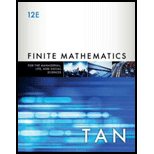# In Exercises 1 − 16 , determine whether the argument is valid. p ↔ q q ∨ r ~ r ∴ p → ~ r### Finite Mathematics for the Manager...

12th Edition
Soo T. Tan
Publisher: Cengage Learning
ISBN: 9781337405782### Finite Mathematics for the Manager...

12th Edition
Soo T. Tan
Publisher: Cengage Learning
ISBN: 9781337405782

#### Solutions

Chapter
Section
Chapter A.5, Problem 14E
Textbook Problem

## In Exercises 1 − 16 , determine whether the argument is valid.   p ↔ q   q ∨ r ~ r                   ∴ p → ~ r

Expert Solution
To determine

To check:

The validity of the given argument pqqr~rp~r

### Explanation of Solution

Approach:

The disjunction is true if at least one of the components of the disjunction is true.

The disjunction is false if both the statements are false.

The conjunction is true if both the components of the conjunction is true.

The conjunction is false if at least one of the statements is false.

If the statement p is true the conditional statement will depend on whether the statement q is true or false.

If the statement p is false the conditional statement will be false and does not depend on whether the statement q is true or false.

The negation p is the opposite of the statement p.

If the component of the statement is true the negation of statement is false and vice-versa.

An argument is valid if the conclusion of the argument is guaranteed under its given set of premises.

An argument having n premises P1,P2,P3,...,Pn and conclusion C can be represented by the conditional [P1P2P3...Pn]C.

Calculation:

Consider the argument pqqr~rp~r

Therefore, the conditional for the argument is,

[(pq)(qr)(~r)](p~r)

There are 3 letters in the given symbolic expression. So, there will be 23=8 rows in the truth table.

There will be eight columns in the truth table

### Want to see the full answer?

Check out a sample textbook solution.See solution

### Want to see this answer and more?

Bartleby provides explanations to thousands of textbook problems written by our experts, many with advanced degrees!

See solution